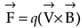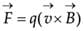# Assertion & Reason Test: Moving Charges & Magnetism - 2

## 10 Questions MCQ Test Topic-wise MCQ Tests for NEET | Assertion & Reason Test: Moving Charges & Magnetism - 2

Description
Attempt Assertion & Reason Test: Moving Charges & Magnetism - 2 | 10 questions in 20 minutes | Mock test for NEET preparation | Free important questions MCQ to study Topic-wise MCQ Tests for NEET for NEET Exam | Download free PDF with solutions
QUESTION: 1

### Directions: In the following questions, A statement of Assertion (A) is followed by a statement of Reason (R). Mark the correct choice as.Assertion (A): If an electron is not deflected when moving through a certain region of space, then the only possibility is that no magnetic field is present in that region.Reason (R): Force on electron is directly proportional to the strength of the magnetic field.

Solution: In absence of magnetic field, moving electrons will not be deflected. This possibility is true. So, the assertion is true.So, force on electrons is directly proportional to the strength of the magnetic field. So, the reason is true. Reason properly explains the assertion.

QUESTION: 2

### Directions: In the following questions, A statement of Assertion (A) is followed by a statement of Reason (R). Mark the correct choice as.Assertion (A): An electron and a proton moving with same velocity enters a magnetic field. The force experienced by the proton is more than the force experienced by the electron.Reason (R): The mass of the proton is more than the mass of the electron.

Solution:So, the force is mass independent. So, the assertion is false. Protons are obviously heavier than electrons. So, the reason is true. But reason does not explain the assertion.

QUESTION: 3

### Directions: In the following questions, A statement of Assertion (A) is followed by a statement of Reason (R). Mark the correct choice as.Assertion (A): The magnetic field produced by a current carrying solenoid is independent of its length and area of cross-section.Reason (R): Magnetic field within a very long solenoid is uniform.

Solution: Magnetic field inside solenoid B = μ0ni. It is independent of length and area of cross-section. Hence the assertion is true. Reason is also true. But it does not explain the assertion.
QUESTION: 4

Directions: In the following questions, A statement of Assertion (A) is followed by a statement of Reason (R). Mark the correct choice as.

Assertion (A): In a moving coil galvanometer, the coil is wound on a metallic frame.

Reason (R): The metallic frame helps in making steady deflection without oscillation.

Solution: Coil of a moving coil galvanometer is wound on a metal frame. So, the assertion is true. It is done to avoid any oscillation and fluctuating reading. The metal frame provides damping to reduce the oscillation so that the reading becomes steady. So the reason is also true and properly explains the assertion.
QUESTION: 5

Directions: In the following questions, A statement of Assertion (A) is followed by a statement of Reason (R). Mark the correct choice as.

Assertion (A): Galvanometer to ammeter conversion takes place by connecting a low value resistance in parallel with it.

Reason (R): The low value resistance increases the effective resistance and protects the galvanometer.

Solution: Galvanometer to ammeter conversion takes place by connecting a low value resistance known as “shunt” in parallel with it. The assertion is true. When two resistors are connected in parallel then the effective resistance becomes lower than the lowest value of the two resistors. Hence the reason is false.
QUESTION: 6

Directions: These questions consist of two statements, each printed as Assertion and Reason. While answering these questions, you are required to choose any one of the following four responses.

Assertion: Cyclotron is a device which is used to accelerate the positive ion.

Reason: Cyclotron frequency depends upon the velocity.

Solution: Cyclotron is a device used to accelerate ions. frequency of it is f = [eB/(2πm)]. This frequency is independent of velocity hence assertion is true and reason is false.
QUESTION: 7

Directions: These questions consist of two statements, each printed as Assertion and Reason. While answering these questions, you are required to choose any one of the following four responses.

Assertion : In electric circuits, wires carrying currents in opposite directions are often twisted together

Reason : If the wires are not twisted together, the combination of the wires forms a current loop, the magnetic field generated by the loop might affect adjacent circuits or components.

Solution: If the wires are twisted together, they can be formed as a single wire carrying currents in opposite directions. In this pattern, in wires no magnetic field is induced which does not affect adjacent circuits.
QUESTION: 8

Directions: These questions consist of two statements, each printed as Assertion and Reason. While answering these questions, you are required to choose any one of the following four responses.

Assertion : A charge, whether stationary or in motion, produces a magnetic field around it.

Reason : Moving charges produce only electric field in the surrounding space.

Solution: A charge whether stationary or in motion produces an electric field around it. If it is in motion then in addition to the electric field it also produces a magnetic field because moving charges produce magnetic field in the surrounding space.
QUESTION: 9

Directions: These questions consist of two statements, each printed as Assertion and Reason. While answering these questions, you are required to choose any one of the following four responses.

Assertion : If the current in a solenoid is reversed in direction while keeping the same magnitude, the magnetic field energy stored in the solenoid remains unchanged.

Reason : Magnetic field energy density is proportional to the magnetic field.

Solution: Reversing the direction of the current reverses the direction of the magnetic field. However, it has no effect on the magnetic-field energy density, which is proportional to the square of the magnitude of the magnetic field.
QUESTION: 10

Directions: These questions consist of two statements, each printed as Assertion and Reason. While answering these questions, you are required to choose any one of the following four responses.

Assertion: To convert a galvanometer into an ammeter a small resistance is connected in parallel with it.

Reason: The small resistance increases the combined resistance of the combination.

Solution: A galvanometer can be converted into an ammeter by connecting a low resistance (called shunt resistance) in parallel to the galvanometer. Parallel resistance being small will draw most of the current and the combination will not effect the original current in the circuit.Use Code STAYHOME200 and get INR 200 additional OFF Use Coupon Code

### How to Prepare for NEET

Read our guide to prepare for NEET which is created by Toppers & the best Teachers